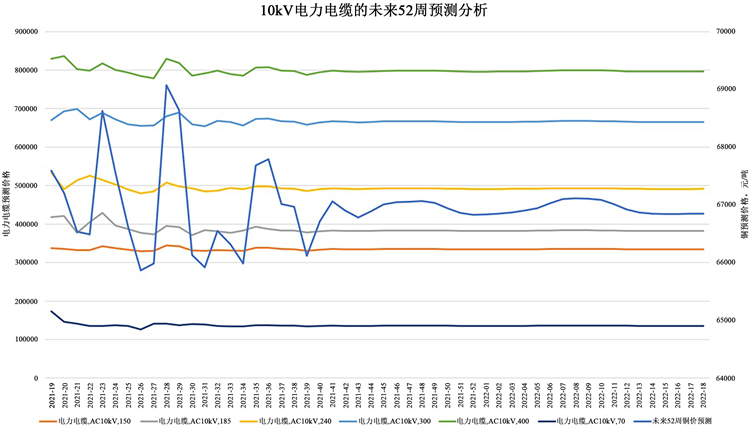#### 期刊菜单

Research on Purchase Price of Finished Materials Based on Neural Network
DOI: 10.12677/PM.2023.131010, PDF, HTML, XML, 下载: 52  浏览: 80

Abstract: Under the dual background of the digital transformation of the energy industry and the current international raw material price fluctuations, the price risk of power enterprises’ material pro-curement is relatively large. In order to reduce the business risks of enterprises and ensure the safety of regional electricity consumption, power enterprises have constructed multi-factor and spatio-temporal features based extraction methods. The time series model predicts the interna-tional copper price. On this basis, the relationship between the raw material and the finished product bidding price is constructed based on the regression model, so as to strengthen the control and decision-making analysis of the material purchase price, and realize the estimation of the reasonable bidding purchase price, trend prediction of future material purchase price and bidding price range setting, so as to improve resource utilization efficiency and ensure the quality and ef-ficiency of material supply.

1. 背景

“2030年碳达峰”“2060年碳中和”目标的提出让中国能源革命有了更明确的时间表，而能源互联网是落实能源革命的具体载体 。为了应对能源转型给电力系统带来的挑战，电力企业坚持聚焦能源消费革命、提升社会综合能效水平，着力推进开源开放、实现经营发展方式转变，推动公司从重资产模式物流企业向现代轻模式平台企业的顺利转型。

1) 电力企业进行招标采购价格申报时，缺乏对未来物资价格趋势预测，往往直接使用物资当前联动价格，导致出现框架协议采购金额低估或超额的现象发生。

2) 招投标过程中智能化程度欠缺，尚未有效运用大数据分析与挖掘的手段匡算物资合理价格区间，缺乏预警防范、供应商不合理报价导致超额利润或低价抢标等现象的有利手段，防范廉政风险能力有待提升。

3) 尽管应用价格联动公式，但原料价格波动巨大，造成采购价格与当前市场价格匹配性不高，导致供应商不愿意履约等不良行为产生，影响物资保障安全。

2. 研究方法

2.1. 多因素和基于时空特征提取的时间序列的铜价预测

ELMAN神经网络是一种典型的动态递归神经网络，可用来预测铜价。其基本公式如下：

$y\left(k\right)=g\left({w}^{3}x\left(k\right)\right)$ (1)

$x\left(k\right)=f\left({w}^{1}{x}_{c}\left(k\right)+{w}^{2}\left(u\left(k-1\right)\right)\right)$ (2)

${x}_{c}\left(k\right)=x\left(k-1\right)$ (3)

${u}_{i}={\sum }_{i=1}^{m}{w}_{jk}\cdot {x}^{\text{T}}$ (4)

${h}_{i}=\psi \left(\frac{{u}_{i}-{b}_{i}}{{a}_{i}}\right)$ (5)

$y={\sum }_{i=1}^{m}{w}_{jk}\cdot {h}_{i}$ (6)

${f}_{t}=\sigma \left({W}_{f}\cdot \left[{h}_{t-1},{x}_{t}\right]+{b}_{f}\right)$ (7)

${i}_{t}=\sigma \left({W}_{i}\cdot \left[{h}_{t-1},{x}_{t}\right]+{b}_{i}\right)$ (8)

$\stackrel{˜}{C}=\mathrm{tanh}\left({W}_{C}\cdot \left[{h}_{t-1},{x}_{t}\right]+{b}_{C}\right)$ (9)

${C}_{t}={f}_{t}×{C}_{t-1}+{i}_{t}×{\stackrel{˜}{C}}_{t}$ (10)

${o}_{t}=\sigma \left({W}_{o}\left[{h}_{t-1},{x}_{t}\right]+{b}_{o}\right)$ (11)

${h}_{t}={o}_{t}×\mathrm{tanh}\left({C}_{t}\right)$ (12)

${e}_{ij}=\frac{f{\left({x}_{i}\right)}^{\text{T}}g\left({x}_{j}\right)}{\sqrt{{d}_{k}}}$ (13)

${a}_{ij}=\text{softmax}\left({e}_{ij}\right)=\frac{\mathrm{exp}\left({e}_{ij}\right)}{{\sum }_{j}\mathrm{exp}\left({e}_{ij}\right)}$ (14)

$o={a}_{ij}h\left({x}_{i}\right)=\text{softmax}\left({e}_{ij}\right)h\left({x}_{i}\right)$ (15)

2.2. 成品与原材料价格回归分析

$b=\frac{\underset{i=1}{\overset{n}{\sum }}\left({x}_{i}-\stackrel{¯}{x}\right)\left(y-\stackrel{¯}{y}\right)}{\underset{i=1}{\overset{n}{\sum }}{\left({x}_{i}-\stackrel{¯}{x}\right)}^{2}}$ (16)

$a=\stackrel{¯}{y}-b\stackrel{¯}{x}$ (17)

3. 实证研究

3.1. 数据概况

1) 铜现货价格，取1#电解铜(2019/01/02至今，日均价，来源：上海有色网)；

2) 铝现货价格，取A00铝(2019/01/02至今，日均价，来源：上海有色网)；

3) 仓单数量(2019/01/02至今，日期末数量，来源：上海期货交易所)，即仓库中可供交割的商品数量；

4) 人民币兑美元汇率(日，来源：中国银行外汇牌价)；

5) WTI原油期货结算价、布伦特原油期货结算价(日，来源：NYMEX)；

6) 2018~2021年招标数据(来源：内部数据)；

7) 2018~2021年采购履约数据(来源：内部数据)。

3.2. 数据清洗及准备

(1) 数据关联：以框架协议编号、框架协议行项目将采购履约数据与中标数据进行关联。

(2) 数据准备：基于铜、铝现货价格，根据招标日期、匹配日期，分别计算其前1天、前7天、前30天的铜、铝原料均价。

(3) 维度聚合：针对采购履约的关联数据进行维度聚合，颗粒度最细保留到物料描述、需求批次、供应商、招标批次维度的采购价格及其他相关数据。

3.3. 成品价格趋势预测

3.4. k值回归分析

k是代表了单位原材料价格变动对成品价格的影响。基于联动公式 $P={P}_{0}+K\ast \left(B-A\right)$ ，发现 $K=\left(P-{P}_{0}\right)/\left(B-A\right)$ ，即(采购履约价 − 中标价)/(履约时上月原料均价 − 中标时原料价格)，是成品价格波动与原料价格波动的比值。它本身是客观存在的值，由成品物料清单中所需的原材料的数量，供应商相关运营成本等因素决定。准确的k值能根据当前市场的原料价格判断合理的成品价格，也能原料价格的预测指导成品价格的走势。Figure 1. Forecast analysis of 10 kV power cable in the next 52 weeks

1) 1120 AB的k值结果更具代表性

2) 原料均价观测时长对k值影响较低

3) 电力电缆的当前k值普遍偏低

4. 推广前景

  刘华军, 石印, 郭立祥, 乔列成. 新时代的中国能源革命: 历程、成就与展望[J]. 管理世界, 2022, 38(7): 6-24. https://doi.org/10.19744/j.cnki.11-1235/f.2022.0098  王晓敏. 多因素和时间序列下铜价格预测模型的构建及应用[D]: [硕士学位论文]. 西安: 西安建筑科技大学, 2020. https://doi.org/10.27393/d.cnki.gxazu.2020.000726  王阳. 基于小波变换和神经网络的电能质量扰动分析研究[D]: [硕士学位论文]. 武汉: 武汉纺织大学, 2021. https://doi.org/10.27698/d.cnki.gwhxj.2021.000117  安颖坤, 朱永丹. 基于线性回归法和指数平滑法对电力负荷的预测[J]. 电力设备管理, 2021(5): 177-179.  袁铭涓, 孙若莹. 基于LSTM神经网络的大宗农产品价格预测研究[J]. 海峡科技与产业, 2021, 34(11): 43-47+60.